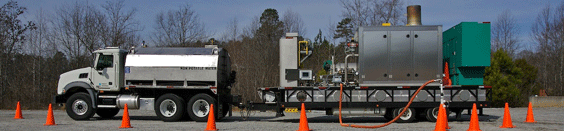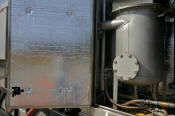Summary of CalculationsDuring the MPE event, a total flow rate measurement of the process stream is taken on the discharge side of the vacuum extraction pump and before off-gas treatment. This measurement is performed using an averaging pitot tube (Dwyer DS‑300) attached to a digital differential pressure sensor. This measurement is used to calculate the removal rates and the off-gas emission rates and is reported in actual cubic feet per minute. A separate flow rate is calculated for the extraction well field, as well as for any additional ambient air introduced into the influent stream. To determine the volume of hydrocarbon removed during the event, samples of the extracted vapors are collected from a sample port located before the vapor stream enters the ThOx unit. A second sample port located on the exhaust stack of the ThOx unit provides access for determining the destruction efficiency of the combustion process.

Concentration measurements are taken using a TVA‑1000A flame ionization detector (FID) calibrated to methane. This FID instrument has a dynamic range of 0 to 50,000 parts per million (PPM) as methane, and 0 to 100,000 PPM as hydrocarbon. The concentration measurements are made before any additional bleed or quench air is added to the extracted vapors; however, these undiluted measurements exceed the dynamic range of the FID instrument. To accurately record the high concentrations commonly observed during an MPE event, a calibrated 10:1 dilution assembly is used to accurately dilute the sample. This dilution assembly, along with the FID instrument, is calibrated before the start of each event.

To account for naturally occurring methane present during a typical MPE event, two concentration measurements are taken. One unfiltered sample measures the total volatile organic compounds (VOCs) in the subsurface. The other sample is collected using an in-line activated carbon filter, which adsorbs the hydrocarbon compounds, leaving only methane. This methane-only result is then subtracted from the total VOC measurement for use in the mass hydrocarbon removal calculation. However, as with any FID instrument, the non-methane organic compound results are recorded as parts per million by volume (PPMv) as if the concentrations were equivalent to methane gas. A conversion from methane to hydrocarbon, and from volume to weight, is necessary to calculate the accurate hydrocarbon removal. By using the TVA‑1000's factory-certified response ratio for various hydrocarbons, the measurements are converted to equivalent hydrocarbon in milligrams per liter (mg/L). For example, a TVA‑1000 FID has an average response ratio of 600 PPMv per mg/L for unleaded gasoline and 200 PPMv per mg/L for diesel. Following is a summary of calculations.

Flow

Q = 128.8 x K x D x SQRT((P x dP) / (T + 460) x Ss)

Where:

Q =

Flow expressed in standard cubic feet per minute

K =

Flow coefficient (provided by Dwyer Instruments, Inc.)

D =

Inside diameter of process line in inches

SQRT =

Square root

P =

Static line pressure

dP =

Differential pressure expressed in inches of water column (WC)

T =

Temperature in degrees Fahrenheit (plus 460 equals degrees Rankine)

Ss =

Specific gravity at 60 degrees Fahrenheit

Conversion of Field Data (PPMv to mg/m3)

C = (PPMv / R) x (1000 L / 1 m3)

Where:

R =

TVA response ratio supplied in The Foxboro Monitor, Volume 3, Issue 1A

(600 PPMv / (mg/L) for gasoline and 200 PPMv / (mg/L) for diesel)

M = Q x C x c

Where:

M =

Q =

Air flow rate

C =

Contaminant concentration (mg/m3)

c =

(1m3 / 35.31 ft ) x (1 lb / 454 x 103) x (60 min / 1 hr) = 3.743 x 10-6

Conversion of Pounds of Hydrocarbon to Equivalent Gallons

Equivalent Gallons = Ss x c

Where:

Ss =

Specific gravity (0.74 = gasoline, 0.84 = diesel fuel)

c =

8.34 lbs/gallon

Home | Services | Company Profile | Testimonials | Contact Us